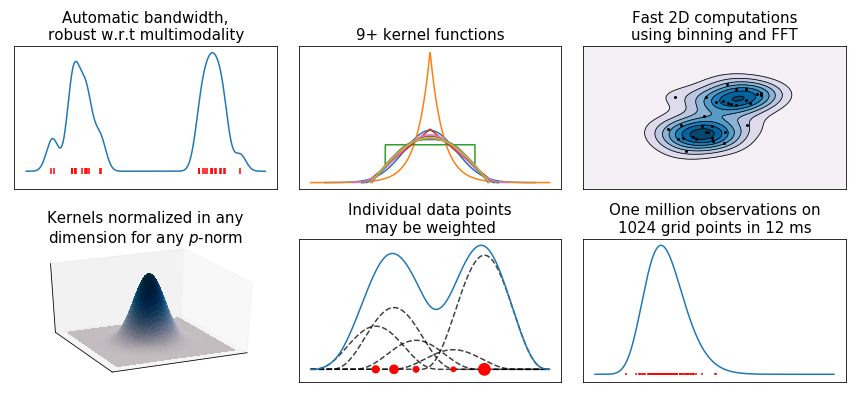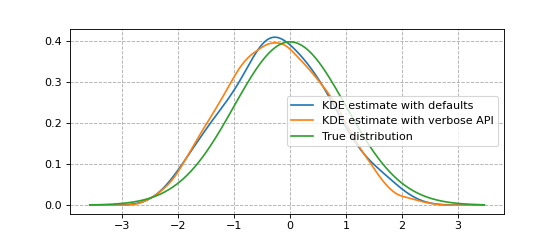# KDEpy¶

This Python 3.5+ package implements various Kernel Density Estimators (KDE). Three algorithms are implemented through the same API: NaiveKDE, TreeKDE and FFTKDE. The FFTKDE outperforms other popular implementations, see the comparison page.The code generating the above graph is found in examples.py.

## Installation¶

KDEpy is available through PyPI, and may be installed using pip:

\$ pip install KDEpy


## Example code¶

Here’s an example showing the usage of FFTKDE, the fastest algorithm implemented. Notice how the kernel and bandwidth are set, and how the weights argument is used. The other classes share this common API of instantiating, fitting and finally evaluating.

from KDEpy import FFTKDE
from scipy.stats import norm

# Generate a distribution and draw 2**6 data points
dist = norm(loc=0, scale=1)
data = dist.rvs(2**6)

# Compute kernel density estimate on a grid using Silverman's rule for bw
x, y1 = FFTKDE(bw="silverman").fit(data).evaluate(2**10)

# Compute a weighted estimate on the same grid, using verbose API
weights = np.arange(len(data)) + 1
estimator = FFTKDE(kernel='biweight', bw='silverman')
y2 = estimator.fit(data, weights=weights).evaluate(x)

plt.plot(x, y1, label='KDE estimate with defaults')
plt.plot(x, y2, label='KDE estimate with verbose API')
plt.plot(x, dist.pdf(x), label='True distribution')
plt.grid(True, ls='--', zorder=-15); plt.legend()The package consists of three algorithms. Here’s a brief explanation:

• NaiveKDE - A naive computation. Supports $$d$$-dimensional data, variable bandwidth, weighted data and many kernel functions. Very slow on large data sets.
• TreeKDE - A tree-based computation. Supports the same features as the naive algorithm, but is faster at the expense of small inaccuracy when using a kernel without finite support. Good for evaluation on non-uniform, arbitrary grids.
• FFTKDE - A very fast convolution-based computation. Supports weighted $$d$$-dimensional data and many kernels, but not variable bandwidth. Must be evaluated on an equidistant grid, the finer the grid the higher the accuracy. Data points may not be outside the grid.

## Issues and contributing¶

If you are having trouble using the package, please let me know by creating an Issue on GitHub and I’ll get back to you. Whatever your mathematical and Python background is, you are very welcome to contribute to KDEpy. To contribute, fork the project on GitHub, create a branch and submit and Pull Request.